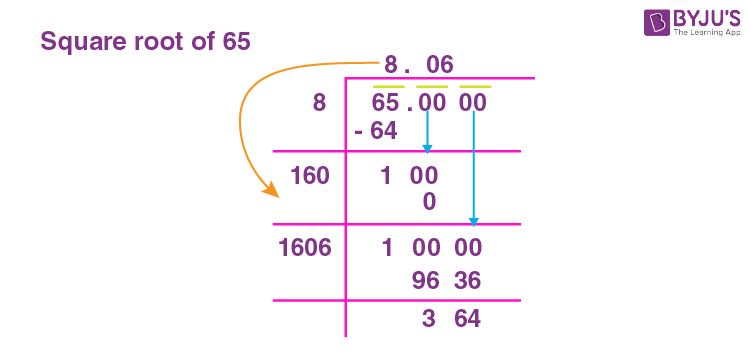# Square Root of 65

The square root of 65 is irrational. The approximate value of the square root of 65 is 8.06.

To determine the square root of 65, one can use methods like:

Let us try the estimation method. The given number is 65. By the list of square and square roots, we know that 8 × 8 = 64 and 9 × 9 = 81. But 65 is just 1 more than 64. This implies that the square root of 65 is just more than 8 and much less than 9, resulting in the decimal root. Steps for the other 3 methods are shown below in this article.

Note the Following:

• The square root of 65 = 2√65 = √65 where ‘√’ is the radical, and 65 is the radicand.
• Exponential Form of Square root of 65 = 651/2
• Solution for √65 ≈ 8.06
• The square root of 65 is Irrational = True.

## What is the Square root of 65?

The square root of 65 is = 8.06, as the square root of 65 is irrational.

 √65 =8.06

## How to Find the Square root of 65?

There are three methods to find the Square root of 65

• Prime Factorisation method
• Long Division method
• Repeated Subtraction method

### Square root of 65 by Prime Factorisation Method

In the prime factorization method, the following steps are followed.

1. Divide 65 with prime factors. The factors are 5, and 13.

Prime factorization of 65 is as follows;

 5 65 13 13 × 1

2. Group 2 same prime factors and remove from the root

3. The product of the numbers outside the root forms the square root.

Since 65 has only 2 factors which are different (5 and 13), the grouping and removal from the root is not possible. Hence, by the prime factorization method, further simplification is not possible.

√65 = (5 × 13)

The long division method can prove the roots more accurately, which is discussed below.

### Square root of 65 by Long Division Method

In the Long Division method, for 65, divide 65 by selecting a divisor such that d × d is less than or equal to 65. If d = 65, then d is the perfect square root of 65, else it is irrational.

Choosing d from the list, the squares are:

7 × 7 = 49

8 × 8 = 64

9 × 9 = 81

10 × 10 = 100Only if the remainder was zero, then the number 65 would have been a perfect square number and would have had a perfect square root. But the remainder is 1 instead of zero. Hence, 65 is not a perfect square. Therefore the square root of 65 is an irrational number.

### Square root of 65 by Repeated Subtraction Method.

In the repeated Subtraction method, 65 is subtracted by odd numbers starting with 1.

The steps to read the below mentioned table for repeated subtraction of the number 65 are:

1. Observe the column 6
2. If 0 is obtained in column 6 in any step, then 65 is a perfect square
3. If a negative number is obtained in column 6 of any step, then 65 is not a perfect square.
4. The step at which the difference is zero, that will be the square root.
 1 2 3 4 5 6 Step 1 65 – 1 = 64 Step 2 64 – 3 = 61 Step 3 61 – 5 = 56 Step 4 56 – 7 = 49 Step 5 49 – 9 = 40 Step 6 40 – 11 = 29 Step 7 29 – 13 = 16 Step 8 16 – 15 = 1 Step 9 1 – 17 = -16

Since the difference is -16 in step 9 and 1 in step 8, the square root 56 is irrational. An approximate decimal number between 8 and 9 forms the root of 56.

## Video Lessons

### Visualising square roots### Finding Square roots## Solved Examples

1. What needs to be subtracted from 65 to get a perfect square number?

Solution: 1 needs to be subtracted from 65 to get 64 which is a perfect square number. Its square root is 8.

2. 65 has a perfect square root number. True or false?

Solution: False. 65 does not have a perfect square root.

## Frequently Asked Questions on Square root of 65

### What is the Square root of 65?

The Square root of 65 is approximately 8.06

### 65 is a perfect square number. True or False?

False. 65 is not a perfect square number.

### What are the prime factors of 65?

The prime factors of 65 are 5 and 13.

### Simplify √65?

√65 = √(5 × 13). No further simplification is possible.

### The square root of 65 is an irrational number. True or False?

True. The square root of 65 is an irrational number.